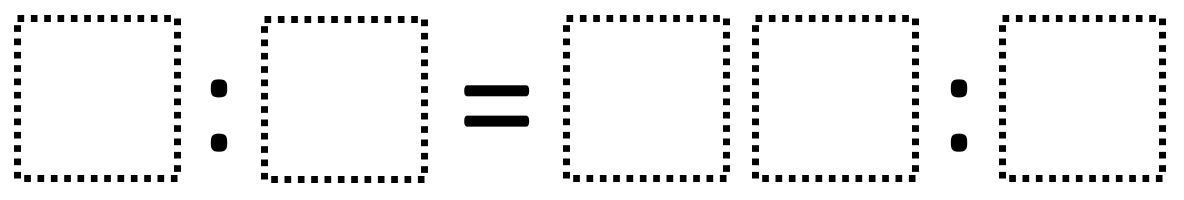# Ratios 1

Directions: Using the digits 0 to 9 at most one time each, fill in the boxes to make an equivalent ratio.### Hint

How can you tell when two ratios are equivalent?

There are many possible answers including 4:1 = 20:5.

Source: Robert Kaplinsky

## Ratios 2

Directions: Using the digits 0 to 9 at most one time each, fill in the …

### One comment

1.this work is so easy when i am in 6th grade i fing math easy because it is the best work i like to do math it is the easiest thing to do.